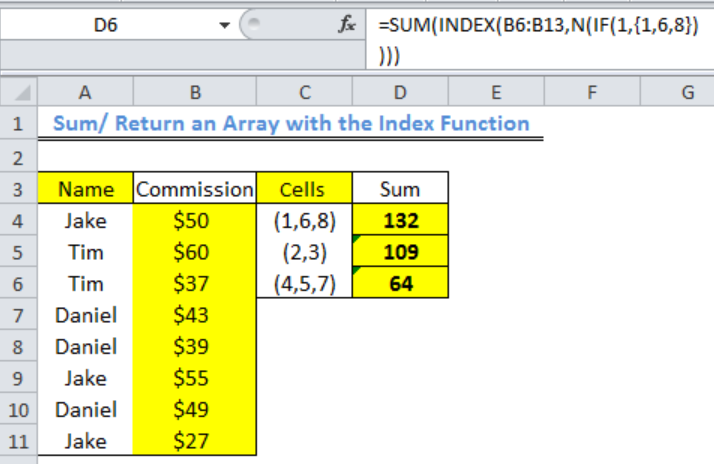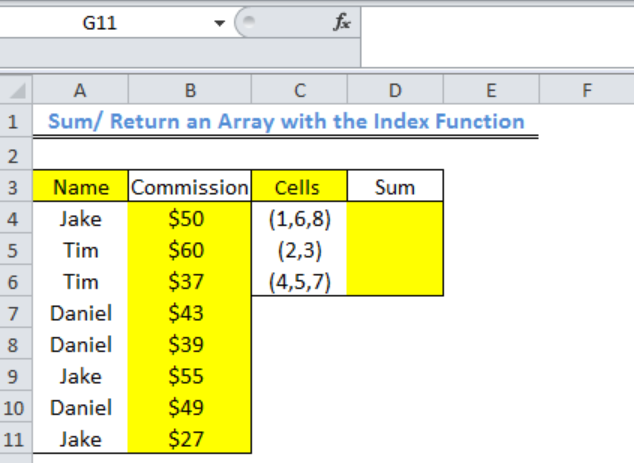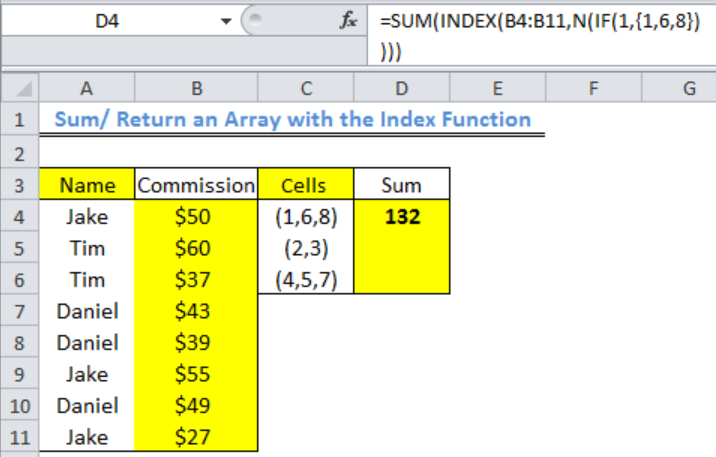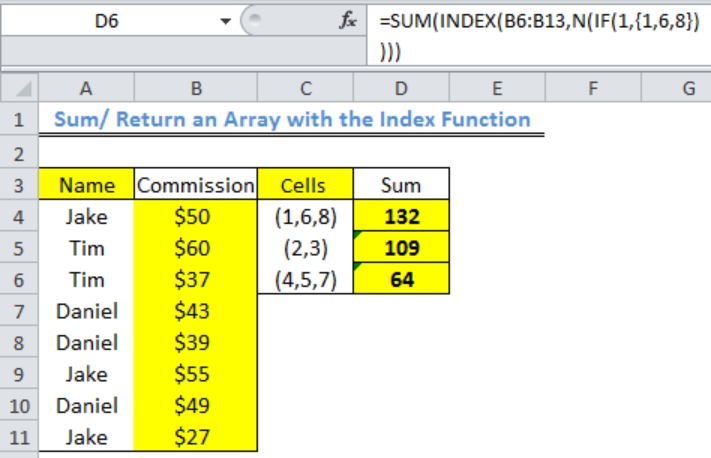Get instant live expert help with Excel or Google Sheets“My Excelchat expert helped me in less than 20 minutes, saving me what would have been 5 hours of work!”

#### Post your problem and you’ll get expert help in seconds.

Your message must be at least 40 characters
Our professional experts are available now. Your privacy is guaranteed.

# How to Sum/Return an Array with the Index Function

We can use the INDEX function to return an ARRAY of values to another function like the SUM function by integrating INDEX with the IF and N functions. We can use this to SUM values that are present in specific cells. This article will walk through the process.Figure 1: How to Sum/ Return an Array with the Index Function

## Formulato Sum/ Return an Array with the Index Function

`=SUM(INDEX(B4:B11,N(IF(1,{1,6,8}))))`

## Setting up the Data

• The names of salespersons have been placed in Column A as shown in figure 2
• The commission of the salespersons are in Column B for certain sold items
• Column C contains the cell numbers for each salesperson’s commission based on the range: A4:A11. We intend to SUM the commissions of each salesperson by using Column C.Figure 2: Setting up the Data

## Sum/ Return an Array with the Index Function

• We will click on Cell D4
• We will input the formula into Cell D4: =SUM(INDEX(B4:B11,N(IF(1,{1,6,8}))))
• We will press the enter key to get the resultFigure 3: Sum of Jake’s Commission

• We will use the fill handle to drag down and get the result for the other two cellsFigure 4: Sum of the Salespeople’s Commission with the SUM Function

## Explanation

The IF function returns as TRUE any number that is contained in the array ({1,6,8}) after the logical test (1) is true (the logical statement here means that any value that is a digit should be returned as TRUE).

The N function returns the values that are returned as TRUE by the IF function to the INDEX function. The INDEX function uses these values to check through the RANGE OF COMMISION (B4:B11) for their positions within COLUMN B. The INDEX FUNCTION then reads the digits in the format below:

• 1 is returned or read as 50
• 6 is returned or read as 55
• 8 is returned or read as 27

The INDEX FUNCTION then returns these numbers to the SUM FUNCTION. The SUM FUNCTION sums the numbers returned by the INDEX FUNCTION and the result is displayed in Cell D4.

Some common errors that you should avoid when using this formula are leaving out the equal to sign and leaving spaces between characters.

## Instant Connection to an Expert through our Excelchat Service

Most of the time, the problem you will need to solve will be more complex than a simple application of a formula or function. If you want to save hours of research and frustration, try our live Excelchat service! Our Excel Experts are available 24/7 to answer any Excel question you may have. We guarantee a connection within 30 seconds and a customized solution within 20 minutes.

### Did this post not answer your question? Get a solution from connecting with the expert.Another blog reader asked this question today on Excelchat:
Related blogs
Solution examplesI need to find out if the 2018 yearly sales goals were met if the yearly sales were \$25,000 or more using an IF logical function and to set the formula to return a value of YES if met, and NO if not
Solved by A. A. in 18 minsI am trying to put in an IF formula and although it works on all the other excel sheets I've worked on it is impossible to get it started in this new tab. The problem would be : =IF(G9="ENT.PSAB",N9,"") It simply doesn't do anything, when what I want is the debit amount to be repeated in another column with the same amount. Save typing in manually.
Solved by C. J. in 60 minsï?·In cell G1enter Discount, ï?·In cell H1enter DiscountPercent,ï?·In cell I1enter DiscountCost,ï?·In cell J1enter TotalCost, andï?·In cell K1enter Paymentas column headings.
Solved by K. E. in 40 minsHow can I automatically change different cells and the information they display if we select different option from a drop down list?
Solved by V. U. in 59 minsI'm looking to offset from =today(), for example: If cell A13=today(), display cell A20. If cell B13=today(), display cell B20. If cell C13=today(), display cell C20. I want to do this for 365 rows. Is there a simpler way of doing this than a huge nested IF statement?
Solved by K. H. in 34 mins## Subscribe to Excelchat.coAnother blog reader asked this question today on Excelchat: